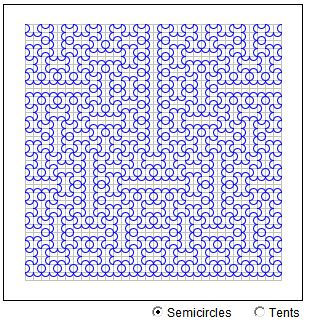# Following the Hilbert Curve

The standard polygonal approximation to the Hilbert plane filling curve is constructed following a simple algorithm that is best expressed in terms of L-Systems [e.g., Chaos and Fractals, Ch. 7]. The standard construction starts with a square U shape. Each step combines four smaller copies of the result of the pervious step: the two middle ones are identical to the previous shape, the other two are obtained by turning the latter left and right.

The applet bellow applies that algorithm to a semicircle and a tent function, instead of the original U shape. Both construction serve exactly the same purpose as the Hilbert curve, viz., filling a square with a continuous curve. In fact, Hilbert himself considered his curve only as a sample resulting from a general procedure described in a 1891 paper.

(To see the progress of polygonal approximations keep clicking in the applet area.)

### If you are reading this, your browser is not set to run Java applets. Try IE11 or Safari and declare the site https://www.cut-the-knot.org as trusted in the Java setup.What if applet does not run?

### Reference

1. Heinz-Otto Peitgen et al, Chaos and Fractals: New Frontiers of Science , Springer, 2nd edition, 2004
2. H. Sagan, Space-Filling Curves, Springer-Verlag, 1994### Plane Filling Curves